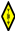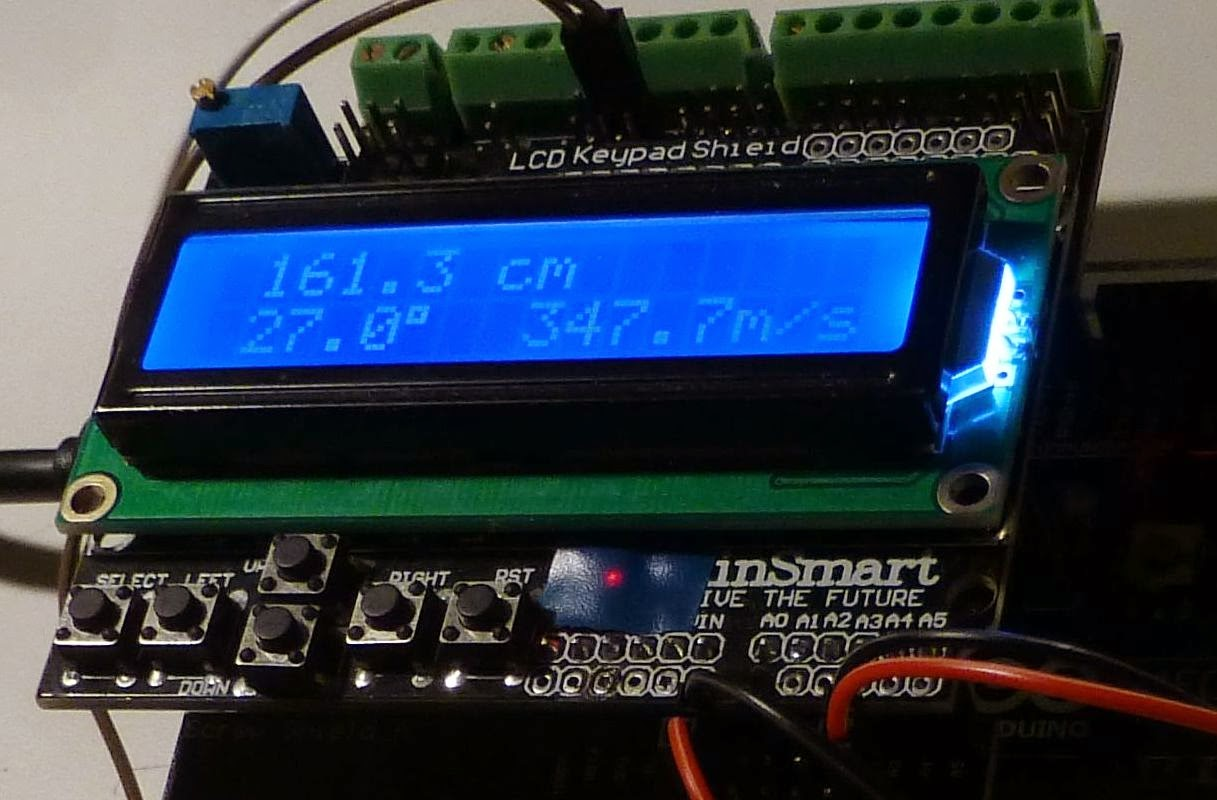##Temperature compensation for an Arduino ultrasonic distance sensor161.3 cm27.0°  347.7 m/s

Ultrasonic distance sensors can find the range out to 2-4 meters and are popular in e.g. robotics. Here I look at how the accuracy can be improved by compensating for the variation of speed of sound with temperature. It actually varies quite a lot in air and around 0 C it is:

c = 331.3 + 0.606 * T

where c is in m/s and T is in C. The formula is good to up to at least +/-30 C. There is also a dependence of humidity, but as it is so small it is neglected here.

The equation can be analyzed for sensitivity (a little bit of differentation, you know). The result is that a two-way range measurement creates an error of 1.8 mm/C/m. That means that with a 4 degree C error, the deviation will be 14.4 mm at a range of 2 meters. Not a lot, but more than the wavelength which is 9 mm at 40 kHz. Considering how easy it is to compensate for, then why not give it a try?

I have tested this with the inexpensive HC-SR04, but the compensation is really independent of the type of sensor. First let me analyze a typical example code for this sensor:

• For range in cm the code divides elapsed time in microseconds by 29. That corresponds to c=10,000/29=344.8 m/s. According to the equation above, this is the speed for a temperature of 22.3 C.
• For range in inches it divides elapsed time by 74. That corresponds to c=1,000,000/74 =13513.5 inches/sec =1126 feet/sec or 343.2 m/sec. This boils down to an assumed temperature of 19.6 C. That’s 2.7 degrees lower than the calculation for cm and means that the measurement in inches is lower than that in cm by 2.7*1.8 = 4.9 mm per meter of range.Temperature is 27.0°, but the program doesn’t use thatvalue and assumes instead its standard value of speed of sound of 344.8 m/s and finds 160.0 cm.

I integrated an HC-SR04 program from Tautvidas Sipavičius with a code from the Arduino playground that uses the DS1820 1-wire temperature sensor (the last program on that page). In the process I had to convert the range estimation from integer to floating point arithmetic. This may give a speed penalty, but at least it runs fine on my Arduino Mega 2560.

The first image in this post shows that at a temperature of 27 C, the speed of sound is 347.7 m/s and the distance from my desk to the ceiling is found to be 161.3 cm. In the picture to the right, I have disabled the temperature compensation so the default velocity of 344.8 m/s is used instead and the estimated distance falls to 160.0 cm.

In the bottom picture, I have detached the 1-wire bus to the temperature sensor, so the program believes it is 0 C, and finds that the range drops to 154.5 cm.154.5 cm 0.0°  331.3 m/s

Now, the HC-SR04 isn’t the most advanced of sensors. Other sensors may have a more accurate detection circuit that works more reliably and with better repeatability at longer ranges. I should also say that since I admitted a digit behind the decimal point in my code that wasn’t there in the original, my measurements wandered a bit from ping to ping also. In reality, I don’t think the HC-SR04 has much more accuracy than 1 cm. But it may have some potential for improvement as e.g shown here: “Making a better HC-SR04 Echo Locator“.

Combining a better detector with compensation for speed of sound variations with temperature should be what it takes to get the ultimate range sensor.

The Arduino sketch measures the range every 0.1 second and pauses for a second every 10 seconds to measure the temperature. The code is here (formatted with Hilite Me):

 `/* Ultrasound distance measurement with compensation for temperature Ultrasound sensor : HC-SR04 Temperature sensor: DS1820 LCD: 2 x 16 lines Created by merging code from http://www.tautvidas.com/blog/2012/08/distance-sensing-with-ultrasonic-sensor-and-arduino/ and http://playground.arduino.cc/Learning/OneWire (Last program on this page) Sverre Holm, 30 May 2014 la3za (a) nrrl.no*/#include #include #include LiquidCrystal lcd(8, 9, 4, 5, 6, 7); #define LCD_WIDTH 16#define LCD_HEIGHT 2#define FIXEDSPEED 0 // turn off temp compensation if == 1/* Ultrasound sensor */int pingPin = 12; int inPin = 13; /* DS18S20 Temperature chip i/o */OneWire ds(24); // (4.7K to Vcc is required)#define MAX_DS1820_SENSORS 1byte addr[MAX_DS1820_SENSORS];int RepeatTemp = 100; // Temp measurement is done every 100*0.1 sec = 10 secvoid setup() { lcd.begin(LCD_WIDTH, LCD_HEIGHT); lcd.print("init ..."); //delay(1000); if (!ds.search(addr)) { lcd.setCursor(0,0); lcd.print("No more addr"); ds.reset_search(); delay(250); return; } if ( !ds.search(addr)) { lcd.setCursor(0,0); lcd.print("No more addr"); ds.reset_search(); delay(250); return; } } int HighByte, LowByte, TReading, SignBit, Tc_100, Whole, Fract;char buf;int cntr = RepeatTemp;void loop() { // *** Part 1: Measure temperature *** // byte i, sensor; byte present = 0; byte data; if (cntr == RepeatTemp) { for ( sensor=0; sensor

Do you like to write?
Interesting project to share?
Helpful tips and ideas for other hams?

Submit an article and we will review it for publication on AmateurRadio.com!

Have a ham radio product or service?

Are you a reporter covering ham radio?

How to Set Up a Ham Radio Blog
Get started in less than 15 minutes!